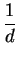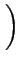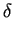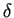Next: 4.2.2.4 Limiting Values Up: 4.2.2 Grid-, Model- and Previous: 4.2.2.2 Scaling of Quantities

### 4.2.2.3 Damping Initialization

To improve the convergence behavior of the total system the update modus of each quantity can be initialized with a start value to use an adaptive damping algorithm that depends on the calculated error of the residual. During the next iterations AMIGOS tries to minimize the defined damping factor depending on the calculated updates of the quantity. The smaller the calculated update the smaller the damping value will be.

Damp(<QuantityName>)= <start value for damping>


To calculate the damping parameter during a Newton iteration the method of Bank and Rose [Ban81] is used:.1 -(4.1)

where d is the damping parameter andvaries between 0 and 1.Next: 4.2.2.4 Limiting Values Up: 4.2.2 Grid-, Model- and Previous: 4.2.2.2 Scaling of Quantities